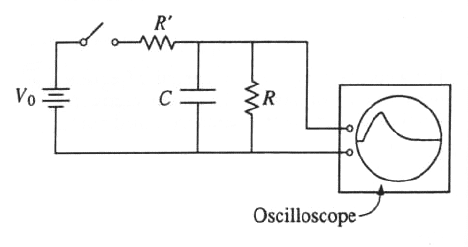GR 8677927796770177 | # Login | Register

GR9277 #86
Problem
 GREPhysics.NET Official Solution Alternate Solutions
\prob{86}THe circuit shown above is used to measure the size of the capacitance C. The y-coordinate of the spot on the oscilloscope screen is proportional to the potential difference across R, and the x-coordinate of the spot is swept at a constant speed s. The switch is closed and then opened. One can then calculate C from the shape and the size of the curve on the screen plus a knowledge of which of the following?

1. and R
2. s and R
3. s and
4. R and R'
5. The sensitivity of the oscilloscope

Lab Methods}Oscilloscopes

The discharge of the capacitor after it has been charged to is just , where . One can find C by knowing and the sweep rate, which is related to t.
(Solution due to David Latchman.)Alternate Solutions
 Herminso2009-09-22 14:33:45 The discharge of the capacitor after it has been charged to is just , thus after the switch is opened, since is in series with the capacitor we need to find , which we can determine by how fast the trace sweeps, s. We need to find , will be given. Thus the answer is (B). The ration can be read off the vertical parts of the scope. Reply to this commentehsana
2013-10-07 17:36:19
Voltage through a discharging capacitor should be .

, as it's written in the solution, is the voltage through a charging capacitor.astro_girl
2013-09-13 06:30:48
The voltage across the capacitor and acrossR, when the switch is closed, is not but . When the switch is closed the capacitor branch is open , but current keeps flowing through R. This doesn't affect the answer though.flyboy621
2010-10-22 21:23:15
You can do this one by elimination.

When the switch is opened, R' is out of the picture, which rules out (D). (E) is too vague to be meaningful, so that choice must be wrong. Finally, it should be obvious that will affect only the scale of the scope display and not the time-behavior of it. Thus (A) and (C) are ruled out. (B) is the only choice left.Herminso
2009-09-22 14:33:45
The discharge of the capacitor after it has been charged to is just

,

thus after the switch is opened, since is in series with the capacitor we need to find , which we can determine by how fast the trace sweeps, s. We need to find , will be given. Thus the answer is (B). The ration can be read off the vertical parts of the scope.oSciL8
2009-03-29 19:04:58
Wait a minute - I thought the time constant (tau = 1/RC) was dependent on the resistor in series with the capacitor? This would mean that one would need to know the sweep rate and R', not R...would someone explain?

Thanks for this website btw - I'll see how helpful its been in a week!!
 gt20092009-06-21 04:13:49 After the switch is opened, R is in series with the capacitor.hamood
2007-04-05 14:42:14
Capacitor discharging is V = Vo
Capacitor charging is V(t) = Vo(1-)
 grace2010-11-10 22:50:28 I agree with you.LaTeX syntax supported through dollar sign wrappers $, ex.,$\alpha^2_0$produces . type this... to get...$\int_0^\infty\partial\Rightarrow\ddot{x},\dot{x}\sqrt{z}\langle my \rangle\left( abacadabra \right)_{me}\vec{E}\frac{a}{b}\$